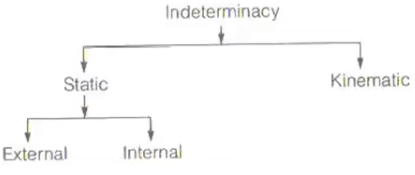Courses

# Determinacy & Indeterminacy of Structures Notes | EduRev

## GATE : Determinacy & Indeterminacy of Structures Notes | EduRev

The document Determinacy & Indeterminacy of Structures Notes | EduRev is a part of the GATE Course Structural Analysis.
All you need of GATE at this link: GATE

Static and Kinematic Indeterminacy

1. Statically Determinate Structures
Conditions of equilibrium are sufficient to analyse the structure. Bending moment and shear force is independent of the cross-sectional area of the components and flexural rigidity of the members. No stresses are caused due to temperature change. No stresses are caused due to lack of fit or differential settlement.
2. Statically Indeterminate Structures
Additional compatibility conditions are required. Bending moment and shear force depends upon the cross-sectional area and flexural rigidity of the members. Stresses are caused due to temperature variation. Stresses are caused due to lack of fit or differential settlement.3. Static Indeterminacy
If a structure cannot be analyzed for external and internal reactions using static equilibrium conditions alone then such a structure is called indeterminate structure.(i) DS = DSe + DSi
Where,
DS = Degree of staticindeterminacy
DSe = External static indeterminacy
DSi = Internal static indeterminacy
External static indeterminacy: It is related with the support system of the structure and it is equal to number of external reaction components in addition to number of static equilibrium equations.
(ii) DSe = re - 3 For 2D
DSe = re – 6 For 3D
Where, re = total external reactions
Internal static indeterminacy: It refers to the geometric stability of the structure. If after knowing the external reactions it is not possible to determine all internal forces/internal reactions using static equilibrium equations alone then the structure is said to be internally indeterminate.
For geometric stability sufficient number of members are required to preserve the shape of rigid body without excessive deformation.
(iii) DSi = 3C - rr …… For 2D
DSi = 6C - r…… For 3D
where, C = number of closed loops.
and
rr = released reaction
(iv) rr = ∑(mj - 1) …… For 2D
rr = 3∑(mj - 1) ……. For 3D
where mj = number of member connecting with J number of joints.
and J = number of hybrid joint.
(v) Ds = m + r­e – 2j ….. For 2D truss
DSe = re - 3 & DSi = m – (2j – 3)
(vi) DS = m + re – 3j ….. For 3D truss
DSe = re – 6 & DSi = m – (3j - 6)
(vii) DS = 3m + re – 3j - rr ….. 2D Rigid frame
(viii) Ds = 6m + r­e – 6j - rr ….. 3D rigid frame
(ix) D= (re – 6) + (6C – rr) ….. 3D rigid frame
4. Kinematic Indeterminacy
It the number of unknown displacement components are greater than the number of compatibility equations, for these structures additional equations based on equilibrium must be written in order to obtain sufficient number of equations for the determination of all the unknown displacement components. The number of these additional equations necessary is known as degree of kinematic indeterminacy or degree of freedom of the structure.
A fixed beam is kinematically determinate and a simply supported beam is kinematically indeterminate.
(i) Each joint of plane pin jointed frame has 2 degree of freedom.
(ii) Each joint of space pin jointed frame has 3 degree of freedom.
(iii) Each joint of plane rigid jointed frame has 3 degree of freedom.
(iv) Each joint of space rigid jointed frame has 6 degree of freedom.
Degree of kinematic indeterminacy is given by:
Dk = 3j - re ………. For 2D Rigid frame when all members are axially extensible.
Dk = 3j - re - m ………. For 2D Rigid frame if 'm' members are axially rigid / inextensible.
Dk = 3(j + j’) - re – m + rr …… For 2D Rigid frame when J' = Number of Hybrid joints is available.
Dk = 6(j + j’) - re – m + rr ….. For 3D Rigid frame
Dk = 2(j + j’) - re – m + rr ….. For 2D Pin jointed truss.
Dk = 3(j + j’) - re – m + rr …… For 3D Pin jointed truss.

Offer running on EduRev: Apply code STAYHOME200 to get INR 200 off on our premium plan EduRev Infinity!

## Structural Analysis

30 videos|122 docs|28 tests

,

,

,

,

,

,

,

,

,

,

,

,

,

,

,

,

,

,

,

,

,

;